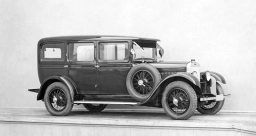# Simultaneously 81403

Cars left two places 520 km apart simultaneously at speeds of 30 km/h and 50 km/h. Where and when will they meet?

s1 =  195 km
s2 =  325 km
t =  6.5 h

### Step-by-step explanation:Did you find an error or inaccuracy? Feel free to write us. Thank you!

Tips for related online calculators
Do you have a linear equation or system of equations and looking for its solution? Or do you have a quadratic equation?
Do you want to convert velocity (speed) units?
Do you want to convert time units like minutes to seconds?